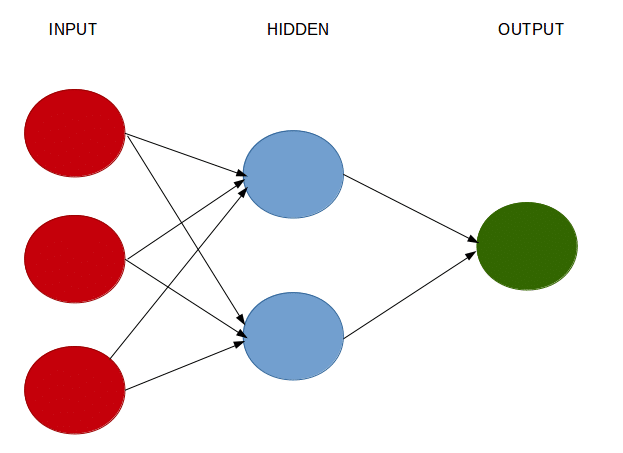# Regression-based neural networks with TensorFlow v2.0In this particular example, a neural network is built in Keras to solve a regression problem, i.e. one where our dependent variable (y) is in interval format and we are trying to predict the quantity of y with as much accuracy as possible.

Keras is an API used for running high-level neural networks — the API is now included as the default one under TensorFlow 2.0, which was developed by Google.

The main competitor to Keras at this point in time is PyTorch, developed by Facebook. While PyTorch has a somewhat higher level of community support, it is a particularly verbose language and I personally prefer Keras for greater simplicity and ease of use in building and deploying models.

In this particular example, a neural network is built in Keras to solve a regression problem, i.e. one where our dependent variable (y) is in interval format and we are trying to predict the quantity of y with as much accuracy as possible.

## Cheat Sheets for AI, Neural Networks, Machine Learning, Deep Learning & Big Data

Cheat Sheets for AI, Neural Networks, Machine Learning, Deep Learning & Big Data

## Learn TensorFlow.js - Deep Learning and Neural Networks with JavaScript

This full course introduces the concept of client-side artificial neural networks. We will learn how to deploy and run models along with full deep learning applications in the browser! To implement this cool capability, we’ll be using TensorFlow.js (TFJS), TensorFlow’s JavaScript library.

## Learning Data Science through competitions

What are the pros and cons of taking Competitive Data Science as a learning path. What is the best way to learn Data Science and Machine Learning?

## Transfer learning for Deep Neural Networks using TensorFlow

A practical and hands-on example to know how to use transfer learning using TensorFlow. We will learn how to use transfer learning for a classification task.

## PySpark in Machine Learning | Data Science | Machine Learning | Python

PySpark in Machine Learning | Data Science | Machine Learning | Python. PySpark is the API of Python to support the framework of Apache Spark. Apache Spark is the component of Hadoop Ecosystem, which is now getting very popular with the big data frameworks.